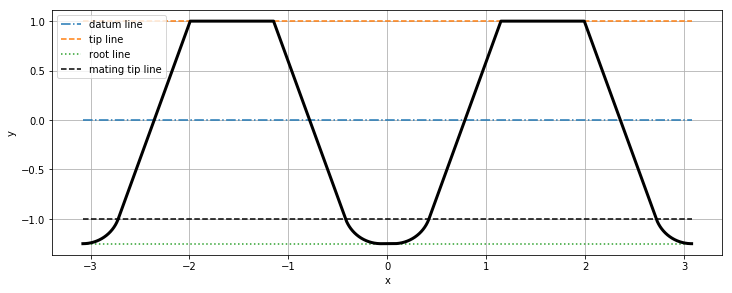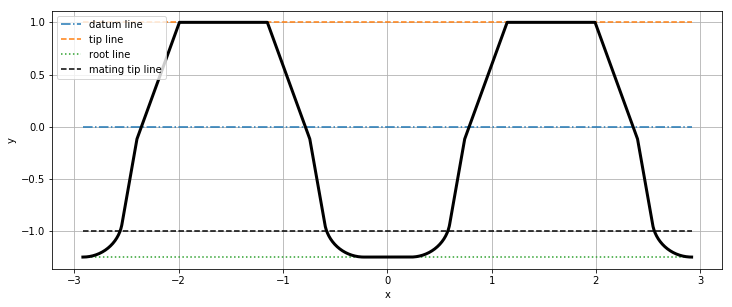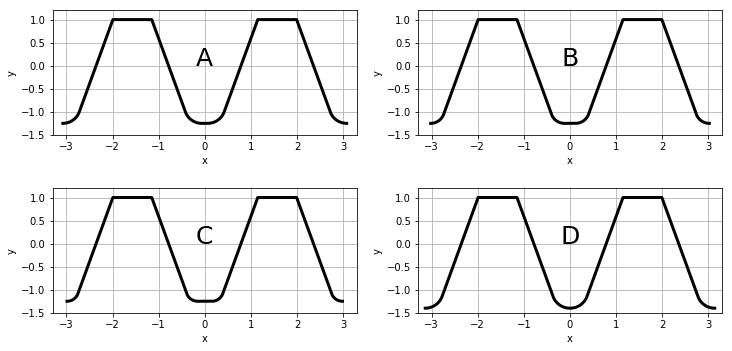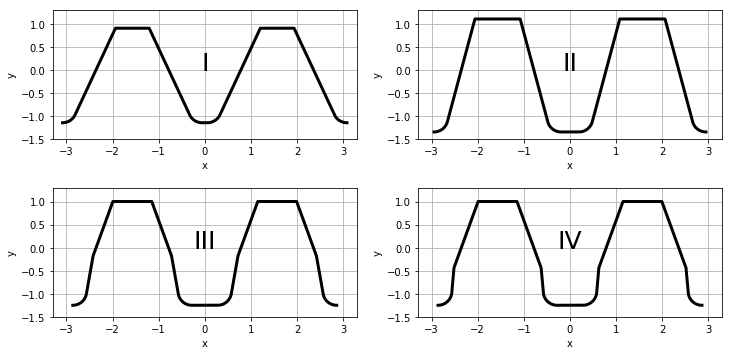# Tooling / Basic Rack¶

Description: Review of basic racks and their significance to manufacturing of cylindrical involute gears.

## Introduction¶

A basic rack defines the tooth profile of a gear with infinite diameter and forms the basis of a family of gears. A basic rack is merely a set of geometric properties for describing gear tooth proportions. It is particularly useful for defining the parameters of a generating cutting tool capable of manufacturing cylindrical involute gears. Later chapters explain such manufacturing methods in detail.

The geometry of a cylindrical involute gear is directly dependent on the manufacturing tooling and processes used to make the gear.

The cost of manufacturing would be prohibitive if every gear required a different tool to make it, hence why the basic rack is of interest to gear manufacturing. Several common methods of gear manufacturing are reviewed in later chapters.

## Geometry¶

The geometry of a basic rack tooth profile is reviewed here.

### Nomenclature¶

Symbol Description
$m_n$ Module
$\alpha_n$ Pressure angle
$p$ Pitch
$p_b$ Base pitch
$h_a$ Addendum
$h_f$ Dedendum
$c$ Bottom clearance
$\rho_F$ Root radius
$\alpha_{FP}$ Undercut angle
$U_{FP}$ Undercut size
$p_{bP}$ Undercut base pitch

Any symbols combined with a superscript asterisk refer to a coefficient, a term normalized by the module. For example, $\rho_F^*$ is the root radius coefficient.Basic rack tooth profile

Notice the geometric definition of a basic rack does not include information about number of gear teeth, gear tooth thickness, tip diameter, or root diameter. This means a given basic rack can be referenced for many possible gear designs, and hence why it serves a good basis for gear tooling.

#### Bottom Clearance¶

Bottom clearance is defined as the distance between the basic rack root line and the tip line of the reciprocal mating rack.Basic rack with mating rack and bottom clearance

As observed in the figure above, the mathematical expression for bottom clearance can be written as:

$$c = h_f - h_a$$

Additionally, the root radius height is equal to the bottom clearance, thus the root radius can be expressed as:

$$\rho_F = \frac{c}{1 - \sin\alpha_n}$$
Rack generation gear cutting tools consider the reciprocal mating rack for tool geometry. In that case, the basic rack root radius represents the tool tip radius, a feature important to the generated shape of a gear tooth root. A deeper understanding of this will be developed in later notebooks.

When designing a gear or its tooling, the maximum root radius of the basic rack is often of interest. The maximum root radius is constrained by the basic rack tooth profile geometry, and may be limited by the bottom clearance to ensure the root radius starts at or below the common tooth depth. The bottom clearance constraint is observed in the previous section.Full root radius of basic rack

If root radius is not limited by bottom clearance, the maximum root radius corresponds to a full root radius. The solution of a full root radius can be obtained by formulating a system of equations. First, an equation can be written for the horizontal coordinate:

$$\rho_F = \frac{s_\text{fillet}}{2 \cos\alpha_n}$$

where $s_\rm{fillet}$ is the spacewidth at the start of the root fillet, an unknown parameter. Next, an equation can be written for the vertical coordinate:

$$\rho_F = \frac{h_\text{fillet}}{1 - \sin\alpha_n}$$

where $h_\text{fillet}$ is the height of the root fillet, an unknown parameter. This equation was applied in the case of a root radius limited by bottom clearance, where $h_\rm{fillet} = c$. We currently have two equations with three unknowns, so we need to introduce additional expressions to create a solvable system of equations. By observing that $s_\text{fillet}$ and $h_\text{fillet}$ can be expressed as functions of a vertical coordinate, $f\{y\}$, we can create a solvable system.

$$s_\text{fillet} = \frac{p}{2} + 2 y_\text{fillet} \tan\alpha_n$$$$h_\text{fillet} = h_f + y_\text{fillet}$$

where $y_\text{fillet}$ is a negative value of the vertical coordinate from the basic rack datum line to the start of the full root fillet. By solving the system of equations, we obtain an expression for $y_\text{fillet}$, which can then be used to find the full root radius.

$$y_\text{fillet} = \frac{e_p \tan\alpha_n - e_p \sec\alpha_n + 2 h_f}{2 (\tan\alpha_n \sec\alpha_n -\tan^2\alpha_n - 1)}$$

where $e_p = \pi m_n / 2$

#### Basic Rack Plot¶

By parametrically defining a basic rack, we can produce a piece-wise plot of the resulting geometry. Below is a such a plot, drawn in the Cartesian xy-plane per the table of input parameters used to define the basic rack.

$m_n$$\alpha_n$$h_a^*$$h_f^*$$\rho_F^*$$\rho_{F,\rm{max}}^*$$p$$p_b$$c^*$
$1\text{mm}$$20^\circ$$1$$1.25$$0.38$$0.38$$3.14\text{mm}$$2.95\text{mm}$$0.25$### Undercut¶

The geometry of an undercut basic rack tooth profile is reviewed here. Undercut is a geometric feature in the root of a basic rack. Undercut exists when the rack tooth profile is not tangent to the root geometry, but instead intersects a line oriented at a pressure angle less than the tooth profile pressure angle.

Undercut angle, $\alpha_{FP}$, and undercut size, $U_{FP}$, are used to define the undercut of a basic rack. These are illustrated in the undercut basic rack below.Undercut basic rack tooth profile

Notice the base pitch of the undercut profile, $p_{bP}$, differs from the tooth profile base pitch, $p_b$, despite each profile having the same pitch, $p$. As we will see in the chapters on spur and helical gear geometry, this difference explains why only gears of the same module and reference pressure angle can mesh. However, there are some exceptions for the generating tool geometry that will be discussed in the relevant notebooks.

Undercut is of particular interest when using a basic rack as a basis for a generating cutting tool, in which case it is referred to as protuberance. Later notebooks on gear tooling will elaborate on the usefulness of protuberance for gear manufacturing and design, and its influence on gear root geometry.

#### Undercut Basic Rack Plot¶

By parametrically defining an undercut basic rack, we can produce a piece-wise plot of the resulting geometry. Below is a such a plot, drawn in the Cartesian xy-plane per the table of input parameters used to define the undercut basic rack.

$m_n$$\alpha_n$$h_a^*$$h_f^*$$\rho_F^*$$\alpha_{FP}$$U_{FP}$
$1\text{mm}$$20^\circ$$1$$1.25$$0.38$$10^\circ$$0.15\text{mm}$## Types¶

There are standard and non-standard basic rack tooth profiles. The standard back rack types have been established by gearing organizations, such as International Standards Organization (ISO) and American Gear Manufacturing Association (AGMA). Gear manufacturers commonly have tools available to cut cylindrical involute gears with the tooth proportions defined by the standard basic rack types.

Depending on the manufacturing method and manufacturer, non-standard basic racks may be used to define the gear tooth proportions. A non-standard basic rack is any basic rack that is not a standard type.

### Standard¶

Standard basic rack types per ISO 53 are defined and illustrated below. Refer to the standard for complete details.

A B C D
Pressure angle, $\alpha_n$ $20^\circ$ $20^\circ$ $20^\circ$ $20^\circ$
Addendum coefficient, $h_a^*$ $1.00$ $1.00$ $1.00$ $1.00$
Dedendum coefficient, $h_f^*$ $1.25$ $1.25$ $1.25$ $1.40$
Root radius coefficient, $\rho_F^*$ $0.38$ $0.30$ $0.25$ $0.39$
Notice the basic rack standard types are only concerned with tooth proportions, not tooth size. This is why module is excluded.### Non-standard¶

Non-standard basic rack tooth profiles are defined and illustrated below. These non-standard racks were arbitrarily defined.

I II III IV
Pressure angle, $\alpha_n$ $25^\circ$ $15^\circ$ $20^\circ$ $20^\circ$
Addendum coefficient, $h_a^*$ $0.90$ $1.10$ $1.00$ $1.00$
Dedendum coefficient, $h_f^*$ $1.15$ $1.35$ $1.25$ $1.25$
Root radius coefficient, $\rho_F^*$ $0.30$ $0.30$ $0.30$ $0.30$
Undercut angle, $\alpha_{FP}$ - - $10^\circ$ $5^\circ$
Undercut size, $U_{FP}$ - - $0.15$ $0.15$